Next: Electric Potential Up: Gauss' Law Previous: Worked Examples

## Example 4.1: Electric field of a uniformly charged sphere

Question: An insulating sphere of radiuscarries a total chargewhich is uniformly distributed over the volume of the sphere. Use Gauss' law to find the electric field distribution both inside and outside the sphere.

Solution: By symmetry, we expect the electric field generated by a spherically symmetric charge distribution to point radially towards, or away from, the center of the distribution, and to depend only on the radial distancefrom this point. Consider a gaussian surface which is a sphere of radius, centred on the centre of the charge distribution. Gauss' law gives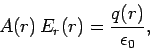where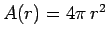is the area of the surface,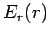the radial electric field-strength at radius, and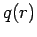the total charge enclosed by the surface. It is easily seen that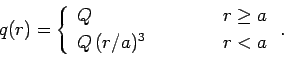Thus,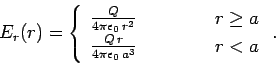Clearly, the electric field-strength is proportional toinside the sphere, but falls off like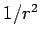outside the sphere.Next: Electric Potential Up: Gauss' Law Previous: Worked Examples
Richard Fitzpatrick 2007-07-14